Next: Stokes Flow Up: Incompressible Viscous Flow Previous: Flow in Slowly-Varying Channels

# Lubrication Theory

It is well known that two solid bodies can slide over one another particularly easily when there is a thin layer of fluid sandwiched between them. Moreover, under certain circumstances, a large positive pressure develops within the layer. This phenomenon is exploited in hydraulic bearings, whose aim is to substitute fluid-solid friction for the much larger friction that acts between solid bodies that are in direct contact with one another. Once set up, the fluid layer in hydraulic bearings offers great resistance to being squeezed out, and is often capable of supporting a useful load.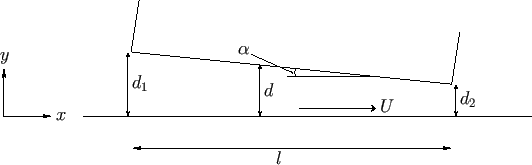Consider the simple two-dimensional case of a solid body with a plane surface (that is almost parallel to the-plane) gliding steadily over another such body, the surface of the gliding body being of finite length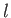in the direction of the motion (the-direction), and of infinite width (in the-direction). (See Figure 10.3.) Experience shows that the plane surfaces need to be slightly inclined to one another. Suppose that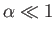is the angle of inclination. Let us transform to a frame of reference in which the upper body is stationary. In this frame, the lower body moves in the-direction at some fixed speed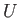. Suppose that the upper body extends fromto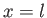, and that the surface of the lower body corresponds to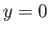. Let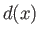be the thickness (in the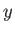-direction) of the fluid layer trapped between the bodies, whereand. It follows that(10.63)

where(10.64)

As discussed in the previous section, provided that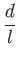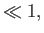(10.65)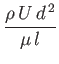(10.66)

the cross-section of the channel between the two bodies is sufficiently slowly varying in the-direction that the channel can be treated as effectively uniform at each point along its length. Thus, it follows from Equation (10.10) that the velocity profile within the channel takes the form(10.67)

where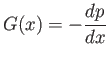(10.68)

is the pressure gradient. Here, we are neglecting gravitational forces with respect to both pressure and viscous forces. The volume flux per unit width (in the-direction) of fluid along the channel is thus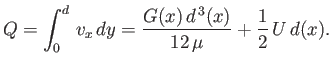(10.69)

Of course, in a steady state, this flux must be independent of. Hence,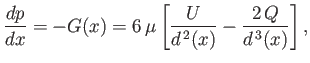(10.70)

where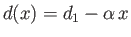. Integration of the previous equation yields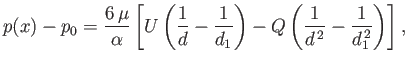(10.71)

where. Assuming that the sliding block is completely immersed in fluid of uniform ambient pressure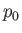, we would expect the pressures at the two ends of the lubricating layer to both equal, which implies that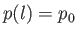. It follows from the previous equation that(10.72)

and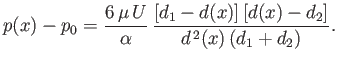(10.73)

Note that if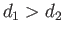then the pressure increment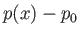is positive throughout the layer, and vice versa. In other words, a lubricating layer sandwiched between two solid bodies in relative motion only generates a positive pressure, that is capable of supporting a normal load, when the motion is such as to drag (by means of viscous stresses) fluid from the wider to the narrower end of the layer. The pressure increment has a single maximum in the layer, and its value at this point is of order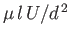, assuming thatis of order unity. This suggests that very large pressures can be set up inside a thin lubricating layer.

The net normal force (per unit width in the-direction) acting on the lower plane is(10.74)

Moreover, the net tangential force (per unit width) acting on the lower plane is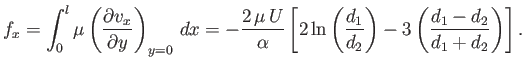(10.75)

Of course, equal and opposite forces,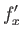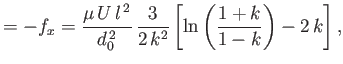(10.76)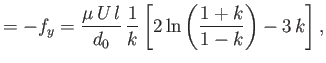(10.77)

act on the upper plane. Here,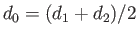is the mean channel width, and. It can be seen that ifthen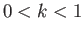. The effective coefficient of friction,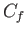, between the two sliding bodies is conventionally defined as the ratio of the tangential to the normal force that they exert on one another. Hence,(10.78)

where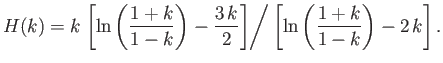(10.79)

The functionis a monotonically decreasing function of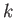in the range. In fact,, whereas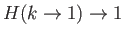. Thus, if[i.e., if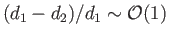] then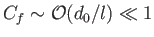. In other words, the effective coefficient of friction between two solid bodies in relative motion that are separated by a thin fluid layer is independent of the fluid viscosity, and much less than unity. This result is significant because the coefficient of friction between two solid bodies in relative motion that are in direct contact with one another is typical of order unity. Hence, the presence of a thin lubricating layer does indeed lead to a large reduction in the frictional drag acting between the bodies.Next: Stokes Flow Up: Incompressible Viscous Flow Previous: Flow in Slowly-Varying Channels
Richard Fitzpatrick 2016-03-31